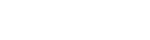# Linear Regression Parameters

Upvote
3
Downvote
Save
Mark Completed
Have you seen this question before?

Given a matrix of X and y values, write a function to generate a transposed matrix and estimate the parameters for linear regression.

Example:

Input:

``````A = [[1, 5], [4,8], [5,9]]

``````

Output:

``````#Output
A_T = [[1, 4, 5], [5, 8, 9]]
coefficients= [4, 1]

#As coefficients is a list of [W0, W1...Wn] as n is the #(number of features).

linear_regression() -> (A_T , coefficients)
``````

Note: Return the solutions a tuple of (`A_T` , `coefficients`)

Next question: Trending Sort
.....